# How to remove text and leave numbers in Excel or vice versa

This tutorial will teach you how to separate text from numbers in Excel by using native formulas and custom functions.

Imagine this: you receive raw data for analysis and find out that numbers are mixed with text in one column. In most situations, it will certainly be more convenient to have them in separate columns for closer examination.

In case you are working with homogeneous data, you could probably use the LEFT, RIGHT, and MID functions to extract the same number of characters from the same position. But that is an ideal scenario for laboratory tests. In real life, you are most likely to deal with dissimilar data where numbers come before text, after text or between text. The below examples provide solutions exactly for this case.

## How to remove text and keep numbers in Excel cells

The solution works in Excel 365, Excel 2021, and Excel 2019

Microsoft Excel 2019 introduced a few new functions that are not available in earlier versions, and we are going to use one of such functions, namely TEXTJOIN, to strip text characters from a cell containing numbers.

The generic formula is:

TEXTJOIN("", TRUE, IFERROR(MID(cell, ROW(INDIRECT( "1:"&LEN(cell))), 1) *1, ""))

In Excel 365 and 2021, this one will also work:

TEXTJOIN("", TRUE, IFERROR(MID(cell, SEQUENCE(LEN(cell)), 1) *1, ""))

At first sight, the formulas may look a bit intimidating, but they do work :)

For example, to remove text from numbers in A2, enter one of the below formulas in B2, and then copy it down to as many cells as needed.

In Excel 365 - 2019:

`=TEXTJOIN("", TRUE, IFERROR(MID(A2, ROW(INDIRECT( "1:"&LEN(A2))), 1) *1, ""))`

In Excel 2019, it must be entered as an array formula with Ctrl + Shift + Enter. In dynamic array Excel, it works as a normal formula completed with the Enter key.

In Excel 365 and 2021:

`=TEXTJOIN("", TRUE, IFERROR(MID(A2, SEQUENCE(LEN(A2)), 1) *1, ""))`

As the result, all text characters are removed from a cell and numbers are kept: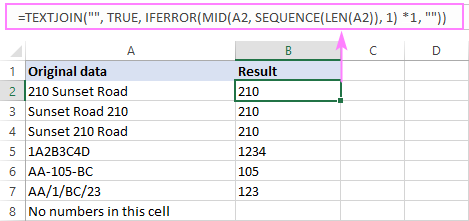How this formula works:

To better understand the logic, let's start investigating the formula from the inside:

You use either ROW(INDIRECT("1:"&LEN(string))) or SEQUENCE(LEN(string)) to create a sequence a numbers corresponding to the total number of characters in the source string, and then feed those sequential numbers to the MID function as the starting numbers. In B2, this part of the formula looks as follows:

`MID(A2, {1;2;3;4;5;6;7;8;9;10;11;12;13;14;15}, 1)`

The MID function extracts each character from A2 beginning with the very first one and returns them as an array:

`{"2";"1";"0";" ";"S";"u";"n";"s";"e";"t";" ";"R";"o";"a";"d"}`

This array is multiplied by 1. Numeric values survive with no change, while multiplying a non-numeric character results in a #VALUE! error:

`{2;1;0;#VALUE!;#VALUE!;#VALUE!;#VALUE!;#VALUE!;#VALUE!;#VALUE!;#VALUE!;#VALUE!;#VALUE!;#VALUE!;#VALUE!}`

The IFERROR function handles these errors and replaces them with empty strings:

`{2;1;0;"";"";"";"";"";"";"";"";"";"";"";""}`

This final array is served to the TEXTJOIN function, which concatenates the non-empty values in the array (ignore_empty argument set to TRUE) using an empty string ("") for the delimiter:

`TEXTJOIN("", TRUE, {2;1;0;"";"";"";"";"";"";"";"";"";"";"";""})`

Tip. For Excel 2016 - 2007, a solution also exists, but the formula is far more complex. You can find it in this tutorial: How to extract numbers in Excel.

### Custom function to remove text from numbers

The solution works for all Excel versions

If you are using an older version of Excel or find the above formulas too difficult to remember, nothing prevents you from creating your own function with a simpler syntax and a user-friendly name such as RemoveText. The user-defined function (UDF) can be written in two ways:

VBA code 1:

Here, we look at each character in the source string one by one and check if it's numeric or not. If a number, the character is added to the resulting string.

VBA code 2:

The code creates an object to process a regular expression. Using RegExp, we remove all characters other than digits 0-9 from the source string.

On small worksheets, both codes will perform equally well. On large worksheets where the function is called hundreds or thousands of times, code 2 that uses VBScript.RegExp will work faster.

The detailed steps to insert the code in your workbook can be found here: How to insert VBA code in Excel.

Whichever approach you choose, from the end-user perspective, the function to delete text and leave numbers is as simple as this:

RemoveText(string)

For instance, to remove non-numeric characters from cell A2, the formula in B2 is:

`=RemoveText(A2)`

Just copy it down the column, and you'll get this result: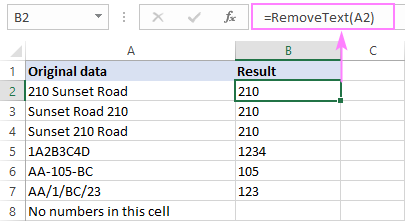Note. Both the native formulas and custom function output a numeric string. To turn it into a number, multiply the result by 1, or add zero, or wrap the formula in the VALUE function. For example:

`=RemoveText(A2) + 0`

`=VALUE(RemoveText(A2))`

## How to remove numbers from text string in Excel

The solution works in Excel 365, Excel 2021, and Excel 2019

The formulas to remove numbers from an alphanumeric string are pretty much similar to the ones discussed in the previous example.

For Excel 365 - 2019:

TEXTJOIN("", TRUE, IF(ISERR(MID(cell, ROW(INDIRECT( "1:"&LEN(cell) )), 1) *1), MID(cell, ROW(INDIRECT("1:"&LEN(cell))), 1), ""))

In Excel 2019, remember to make it an array formula by pressing the Ctrl + Shift + Enter keys together.

For Excel 365 and 2021:

TEXTJOIN("", TRUE, IF(ISERROR(MID(cell, SEQUENCE(LEN(cell 1) *1), MID(cell, SEQUENCE(LEN(cell)), 1), ""))

For example, to strip numbers from a string in A2, the formula is:

`=TEXTJOIN("", TRUE, IF(ISERR(MID(A2, ROW(INDIRECT( "1:"&LEN(A2) )), 1) *1), MID(A2, ROW(INDIRECT("1:"&LEN(A2))), 1), ""))`

Or

`=TEXTJOIN("", TRUE, IF(ISERROR(MID(A2, SEQUENCE(LEN(A2)), 1) *1), MID(A2, SEQUENCE(LEN(A2)), 1), ""))`

As the result, all numbers are removed from a cell and text characters are kept: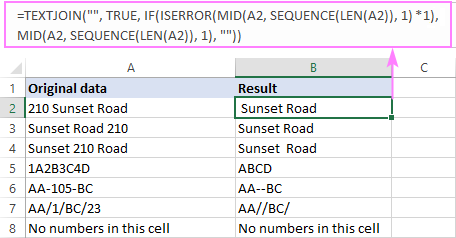As shown in the screenshot above, the formula strips numeric characters from any position in a string: in the beginning, in the end, and in the middle. However, there is a caveat: if a string starts with a number followed by a space, that space is retained, which produces a problem of leading spaces (like in B2).

To get rid of extra spaces before text, wrap the formula in the TRIM function like this:

`=TRIM(TEXTJOIN("", TRUE, IF(ISERROR(MID(A2, SEQUENCE(LEN(A2)), 1) *1), MID(A2, SEQUENCE(LEN(A2)), 1), "")))`

Now, your results are absolutely perfect!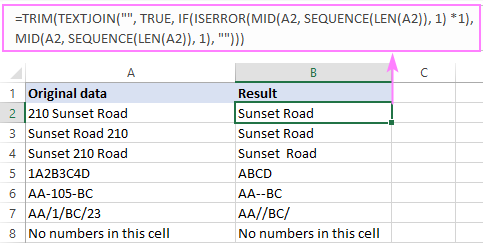How this formula works:

In essence, the formula works the same as explained in the previous example. The difference is that, from the final array served to the TEXTJOIN function, you need to remove numbers, not text. To have it done, we use the combination of IF and ISERROR functions.

As you remember, MID(…)+0 generates an array of numbers and #VALUE! errors representing text characters in the same positions:

`{2;1;0;#VALUE!;#VALUE!;#VALUE!;#VALUE!;#VALUE!;#VALUE!;#VALUE!;#VALUE!;#VALUE!;#VALUE!;#VALUE!;#VALUE!}`

The ISERROR function catches the errors and passes the resulting array of Boolean values to IF:

`{FALSE;FALSE;FALSE;TRUE;TRUE;TRUE;TRUE;TRUE;TRUE;TRUE;TRUE;TRUE;TRUE;TRUE;TRUE}`

When the IF function sees TRUE (an error), it inserts the corresponding text character into the processed array with the help of another MID function. When the IF function sees FALSE (a number), it replaces it with an empty string:

`{"";"";"";" ";"S";"u";"n";"s";"e";"t";" ";"R";"o";"a";"d"}`

This final array is passed over to TEXTJOIN, so it concatenates the text characters and outputs the result.

### Custom function to remove numbers from text

The solution works for all Excel versions

Keeping in mind that a robust formula should be kept simple, I will share the code of the user-defined function (UDF) to strip off any numeric character.

VBA code 1:

VBA code 2:

As is the case with the RemoveText function, the second code is better to be used in large worksheets to optimize the performance.

Once the code is added to your workbook, you can remove all numeric characters from a cell using this custom function:

RemoveNumbers(string)

In our case, the formula in B2 is:

`=RemoveNumbers(A2)`

To trim leading spaces if any, nest the custom function inside TRIM like you would a native formula:

`=TRIM(RemoveNumbers(A2))`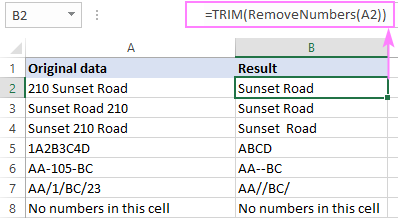## Split numbers and text into separate columns

In situation when you want to separate text and numbers into two columns, it would be nice to have the job done with a single formula, agree? For this, we just merge the code of the RemoveText and RemoveNumbers functions into one function, named SplitTextNumbers, or simply Split, or whatever you like :)

VBA code 1:

VBA code 2:

Our new custom function requires two arguments:

SplitTextNumbers(string, is_remove_text)

Where is_remove_text is a Boolean value indicating which characters to strip:

• TRUE or 1 - remove text and keep numbers
• FALSE or 0 - remove numbers and keep text

For our sample dataset, the formulas take this form:

To remove non-numeric characters:

`=SplitTextNumbers(A2, TRUE)`

To delete numeric characters:

`=SplitTextNumbers(A2, FALSE)`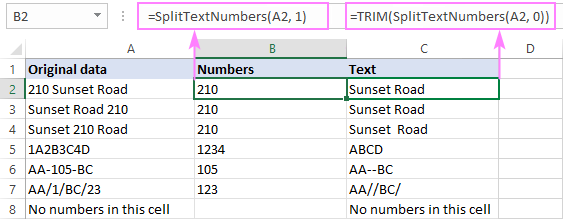Tip. To avoid a potential problem of leading spaces, I recommend always wrapping the formula that removes numbers in the TRIM function:

`=TRIM(SplitTextNumbers(A2, FALSE))`

## Special tool to remove numbers or text

For those who do not like complicating things unnecessarily, I'll show our own way of removing text or numbers in Excel.

Assuming our Ultimate Suite is added to your Excel ribbon, this is what you do:

1. On the Ablebits Data tab, in the Text group, click Remove > Remove Characters.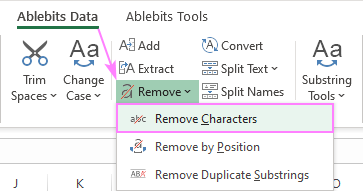2. On the add-in's pane, select the source range, choose the Remove character sets option, and pick either Text characters or Numeric characters in the drop-down list.
3. Hit Remove and enjoy the result :)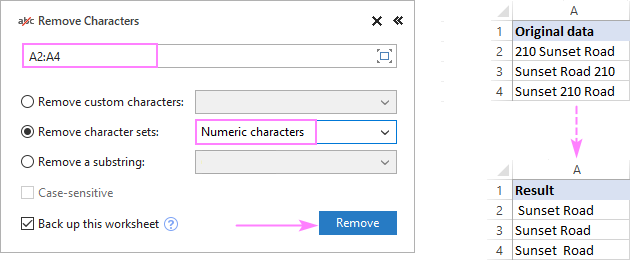Tip. If the results contain some leading spaces, the Trim Spaces tool will eliminate them in no time.

That's how to remove text or numeric characters from a string in Excel. I thank you for reading and look forward to seeing you on our blog next week!